# Excel Add months to date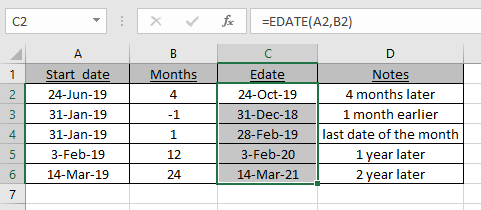Scenario:

If you want to add months to a date in excel, you can’t just simply do addition to add months. Let's assume If you add some months as a number to a date it will actually add days. If you add multiples of 30 (30 day = 1 month) to date then it will give incorrect answers as you don't know to add 30, 31, 28 or even 29.. To add months to a date in excel, let's use excel functions to read date values and extract required results.

Add months Formula using EDATE function in Excel

EDATE function in excel returns the serial number of the date after adding the number of months to a given date.

Formula syntax

 =EDATE(start_date,num_of_months)

Start_date: the date in which you want to add months.

Num_of_months: The number of months you want to add in start date.

Add months Formula using DATE function in Excel

We extracted year, month and date from the date using the respective excel function of the same name. Added 5 months  MONTH(A2)+5. And then integrated year, month and date using the DATE function of excel. This gave us a new date of 5 months ahead.

Formula syntax

 =DATE(YEAR(date), MONTH(date)+ months ,DAY(date))

date : the date in which you want to add years.

months : the number of years you want to add.

Example :

All of these might be confusing to understand. Let's understand how to use the function using an example. Here we have some dates and we need to find the Date value for the given dates and months.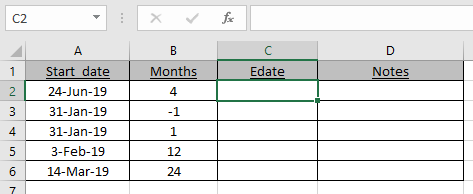Use the formula:

 =EDATE(A2,B2)

A2 : Given date

B2 : number of months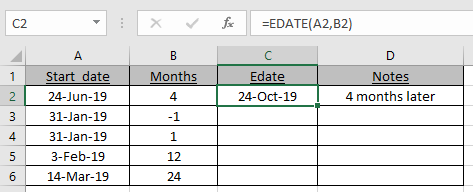As you can see the new_date or EDATE is here.

Now use the formula to the remaining cells to get the EDATE for a given date.As you can see in C4 cell, 31st Jan becomes 28th Feb as 28th is the last date of the Feb.

EDATE formula gets you the date of expiry, maturity dates or other due dates.

For this example we have this data, in A2 there is a start date, in B2 a number of months to add and in C2 we will write a formula for adding months to this date.

Write this formula in C2

 =EDATE(A2,B2)

This will return the serial number of date which is not much readable for a normal user.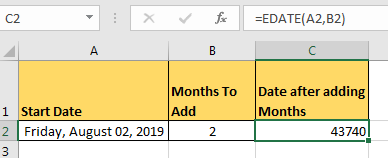To make it readable change the text format to date of cell C2. You can copy format from cell A2 and paste it on C2. whichever way you like, you do it yourself.

Finally you’ll have this readable date.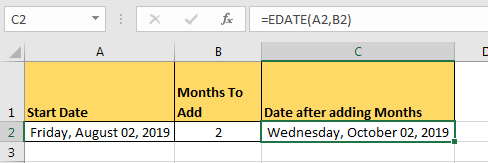## How to subtract months from a date

To subtract months just add minus sign before number of months,

 =EDATE(A2,-B2)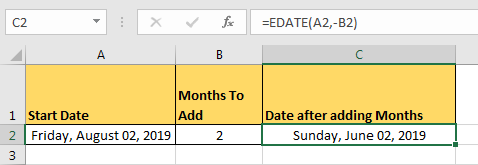## How to Add Years in a Date

As we all know that each year has 12 months in it. So that’s it. In the EDATE function, at the place of num_of_months write the number of years and multiply it by 12.

For example if I want to add 2 year in Friday, August 02, 2019 i will write this formula to add years in excel.

Use the formula:

 =EDATE(A2,B2*12)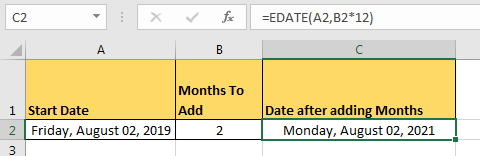Same goes for subtracting years from a date.

Here are all the observational notes using the formula in Excel
Notes :

1. Excel stores dates as serial numbers and is used in calculation by the function. So it’s recommended to use dates as cell reference or using the DATE function instead of giving direct argument to the function.
2. Check the validation of date values if the function returns #VALUE! Error.
3. If the start date is greater than the end date, the function returns the number of days with a negative sign.
4. If the start date and end date is the same date and that date is working, the function returns 1.
5.  If the start date or end date is out of range, then function returns #NUM! Error.
6. Arguments must be given as array reference, not the individual dates separated using commas, the function throws too many arguments error.

Hope this article about How to Excel Add months to date is explanatory. Find more articles on calculating values and related Excel formulas here. If you liked our blogs, share it with your friends on Facebook. And also you can follow us on Twitter and Facebook. We would love to hear from you, do let us know how we can improve, complement or innovate our work and make it better for you. Write to us at info@exceltip.com.

Related Articles :

Extract days between dates ignoring years in Excel : count the days between the two dates using different functions and mathematical operation in Excel.

Count holidays between dates in Excel : count non working days between the two given dates using the DATEDIF and NETWORKDAYS function in Excel.

How to Convert date to text in Excel : In this article we learned how to convert text into date, but how do you convert an excel date into text. To convert an excel date into text we have a few techniques.

Count Birth Dates By Month in Excel : count the number of dates lying in a particular month using the SUMPRODUCT and MONTH function in Excel.

How to use the NETWORKDAYS function in Excel : Returns the working days between the two given dates using the NETWORKDAYS function.

Popular Articles :